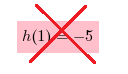### Home > CCA2 > Chapter 1 > Lesson 1.1.1 > Problem1-5

1-5.

Angelica is working with function machines. She has the two machines $g\left(x\right)=\sqrt{x-5}$ and $h(x)=x^2−6$. She wants to put them in order so that the output of the first machine becomes the input of the second. She wants to use a beginning input of $6$.

1. In what order must she put the machines to get a final output of 5?

Try substituting $6$ for $x$ in $g(x)$ and then input the result into $h(x)$. If that does not work, switch the order.

Substitute $6$ for each $x$.

$g(6)=\sqrt{6-5}$

Simplify.

$g(6)=1$

Substitute the output of the first function for $x$ in the second function.

$h(1)=1^{2}-6$

The output needs to be $5$.2. Is it possible for her to get a final output of -5? If so, show how she could do that. If not, explain why not.

See above (original incorrect solution).